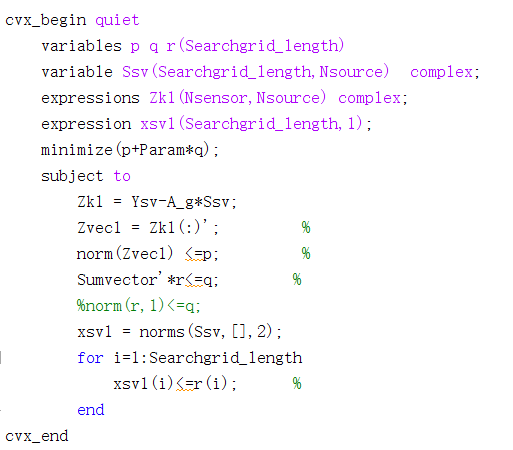# How to solve SOCP problem that contains complex values

I am sorry that I am asking this problem in the forum, but I hope it will work.
I am working on a SOCP problem by CVX recently. It works very well in MATLAB.
However, I need to transform the codes into C++ codes so I was stuck in the step that calculates the SOCP problem. Since there are many solvers for C++ to solve SOCP problem like Gurobi, MOSEK, ECOS, etc, I tried ECOS but the results showed ECOS cannot support complex values since the problem is kind of signal processing problem.
If possible, can someone give me some suggest on how to sort out this problem? I really have been troubled by a long time.
The MATLAB code is as follows:This is not a question about CVX. Express all your complex variables as a+bi, do the little bit of math and rewrite all constraints in terms of that. That’s what CVX does behind the scenes for you.